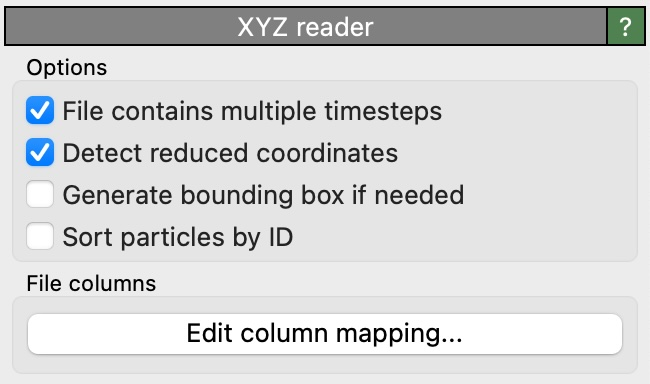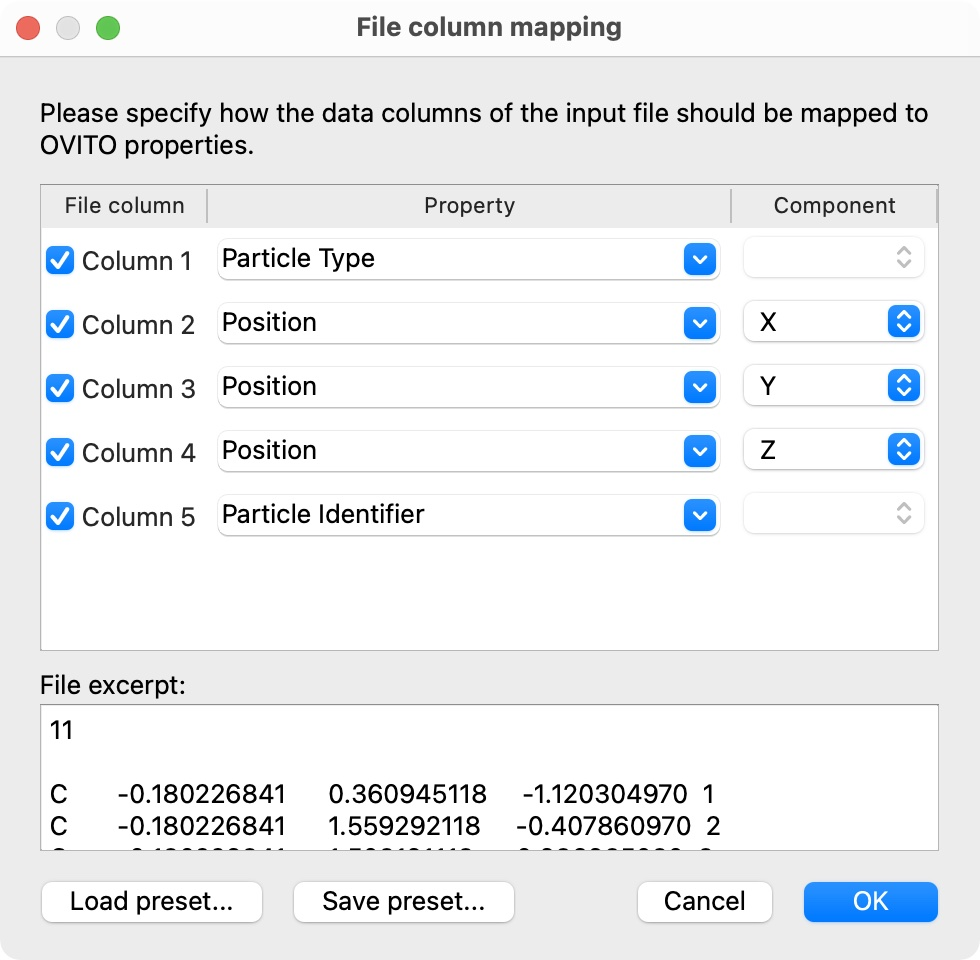# XYZ file readerUser interface of the XYZ reader, which appears as part of a pipeline’s file source.

XYZ is a simple text-based file format for storing particles or atoms and their properties.

## Basic XYZ format

In its simplest form, an XYZ file consists of a short header (two lines) followed by the list of atoms:

8
Cubic bulk silicon cell
Si        0.00000000      0.00000000      0.00000000
Si        1.36000000      1.36000000      1.36000000
Si        2.72000000      2.72000000      0.00000000
Si        4.08000000      4.08000000      1.36000000
Si        2.72000000      0.00000000      2.72000000
Si        4.08000000      1.36000000      4.08000000
Si        0.00000000      2.72000000      2.72000000
Si        1.36000000      4.08000000      4.08000000


The first line specifies the number of atoms and the second line is used to store an arbitrary comment text (may be an empty line). Each of the following atom lines consist of the species name and the atom’s Cartesian xyz coordinates.

Note

Since this basic XYZ file format doesn’t contain any information about the simulation cell, OVITO automatically computes an ad-hoc bounding box enclosing all atoms and assumes non-periodic boundary conditions. This tight simulation box will typically not reflect the actual simulation cell used in the original simulation. If possible, use the extended XYZ file format to import the true cell geometry into OVITO.

This reader is able to load gzipped XYZ files (“.gz” suffix).

XYZ files may store simulation trajectories. Multiple frames are simply stored back-to-back in one file, i.e., the next two-line header directly follows after the atoms list of the preceding frame. OVITO automatically detects if the loaded XYZ file contains more than one frame.

## XYZ files with additional columns

While the basic XYZ format consists of exactly four data columns as described above, OVITO is prepared to read XYZ files with an arbitrary number of columns containing auxiliary per-atom attributes. The file reader will display the following dialog window to let you specify the mapping of each file column to a corresponding particle property within OVITO.OVITO normally adopts the original order of the atoms as they are listed in the XYZ file. However, if a file column contains unique atom IDs, and they are mapped to OVITO’s Particle Identifier property, the file reader provides a user option to sort atoms by ID during import (sort_particles keyword parameter in Python, see below).

## Extended XYZ format

The extended XYZ format is an enhanced version of the basic XYZ format, allowing extra columns to be present in the file for additional per-atom properties as well as standardizing the format of the comment line to include the simulation cell geometry, boundary conditions, and other per-frame parameters. Here is an example:

8
Lattice="5.44 0.0 0.0 0.0 5.44 0.0 0.0 0.0 5.44" Properties=species:S:1:pos:R:3 Time=0.0
Si        0.00000000      0.00000000      0.00000000
Si        1.36000000      1.36000000      1.36000000
Si        2.72000000      2.72000000      0.00000000
Si        4.08000000      4.08000000      1.36000000
Si        2.72000000      0.00000000      2.72000000
Si        4.08000000      1.36000000      4.08000000
Si        0.00000000      2.72000000      2.72000000
Si        1.36000000      4.08000000      4.08000000


In the extended XYZ format, the comment line is replaced by a series of key/value pairs. The keys should be strings, and values can be integers, reals, booleans (denoted by T and F for true and false) or strings. Quotes are required if a value contains any spaces (like Lattice above). There are two mandatory parameters that any extended XYZ file must specify: Lattice and Properties. Other parameters - e.g. Time in the example above - can be added to the parameter line as needed and will be imported by OVITO as global attributes.

Lattice is a Cartesian 3x3 matrix representation of the simulation cell vectors, with each vector stored as a column and the 9 values listed in Fortran column-major order, i.e. in the form:

Lattice="<ax> <ay> <az> <bx> <by> <bz> <cx> <cy> <cz>"


where $$(a_x\ a_y\ a_z)$$ are the Cartesian x-, y- and z-components of the first simulation cell vector $$\mathbf{a}$$, $$(b_x\ b_y\ b_z)$$ those of the second simulation cell vector $$\mathbf{b}$$, and $$(c_x\ c_y\ c_z)$$ those of the third simulation cell vector $$\mathbf{c}$$. Optionally, the Cartesian coordinates of the simulation cell origin $$\mathbf{o} = (o_x\ o_y\ o_z)$$ can be specified as follows:

Origin="<ox> <oy> <oz>"


The periodic boundary conditions in each cell direction may be specified as triplet of Boolean flags (F/T or 0/1), e.g.:

pbc="T T F"


If the pbc keyword is not present, OVITO assumes the simulation cell to be periodic in all three directions (only if it’s an extended XYZ files including the Lattice keyword!).

The list of data columns in the file is described by the Properties parameter, which should take the form of a series of colon-separated triplets giving the name, format (S for string, R for real, I for integer) and number of columns of each property. For example:

Properties="species:S:1:pos:R:3:vel:R:3:flagged:I:1"


indicates that the first file column represents atomic species, the next three columns represent atomic positions, the next three velocities, and the last is an single integer called flagged. With this columns definition, the line

Si        4.08000000      4.08000000      1.36000000   0.00000000      0.00000000      0.00000000       1


would describe a silicon atom at position $$(4.08, 4.08, 1.36)$$ with zero velocity and the flagged particle property set to 1. In the current version of OVITO, text columns (data format S) are only allowed for the atom species or the molecule type.

The file reader automatically maps file columns to the right particle properties in OVITO if their name matches one of the following standard names (case-insensitive):

XYZ column specification

OVITO particle property

type:I:1

Particle Type

species:S:1

Particle Type

element:S:1

Particle Type

atom_types:I:1

Particle Type

pos:R:3

Position

color:R:3

Color

disp:R:3

Displacement

disp_mag:R:1

Displacement Magnitude

force:R:3

Force

forces:R:3

Force

velo:R:3

Velocity

velo_mag:R:1

Velocity Magnitude

radius:R:1

Radius

id:I:1

Particle Identifier

aspherical_shape:R:3

Aspherical Shape

orientation:R:4

Orientation

map_shift:I:3

Periodic Image

transparency:R:1

Transparency

vector_color:R:3

Vector Color

molecule:I:1

Molecule

molecule_type:S:1

Molecule Type

cluster:I:1

Cluster

n_neighb:I:1

Coordination

structure_type:S:1

Structure Type

stress:R:6

Stress Tensor

strain:R:6

Strain Tensor

deform:R:9

Deformation Gradient

mass:R:1

Mass

charge:R:1

Charge

dipoles:R:3

Dipole Orientation

dipoles_mag:R:1

Dipole Magnitude

omega:R:3

Angular Velocity

angular_momentum:R:3

Angular Momentum

torque:R:3

Torque

spin:R:1

Spin

centro_symmetry:R:1

Centrosymmetry

selection:I:1

Selection

local_energy:R:1

Potential Energy

kinetic_energy:R:1

Kinetic Energy

total_energy:R:1

Total Energy

File columns having any other name will be mapped to a new user-defined particle property of the same name.

## OpenBabel exyz format

OVITO supports also the .exyz format written by OpenBabel, which contains a comment line starting with the token %PBC. In this variant of the XYZ format, the simulation cell geometry follows behind the atoms list as a separate section.

## Python parameters

The XYZ file reader accepts the following optional keyword parameters in a Python call to the import_file() or load() functions.

import_file(location, columns=None, rescale_reduced_coords=True, sort_particles=False, **kwargs)
Parameters
• columns (list[str|None] or None) – A list of OVITO particle property names, one for each data column in the xyz file. Overrides the mapping that otherwise gets set up automatically as described above. List entries may be set to None to skip individual file columns during parsing.

• rescale_reduced_coords – If set to True, and if the extended XYZ file specifies the dimensions of the simulation cell, and if all atomic coordinates are either in the range [0,1] or [-0.5,0.5], the file reader will convert the reduced coordinates to Cartesian coordinates before storing them into the Position particle property.

• sort_particles (bool) – Makes the file reader reorder the loaded particles before passing them to the pipeline. Sorting is based on the values of the Particle Identifier property loaded from the xyz file, if any.